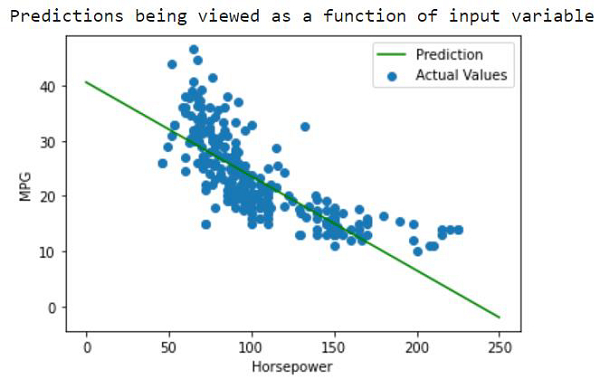# How can predictions be made on Auto MPG dataset using TensorFlow?

Tensorflow is a machine learning framework that is provided by Google. It is an open-source framework used in conjunction with Python to implement algorithms, deep learning applications and much more. It is used in research and for production purposes.

Tensor is a data structure used in TensorFlow. It helps connect edges in a flow diagram. This flow diagram is known as the ‘Data flow graph’. Tensors are nothing but multidimensional array or a list. They can be identified using three main attributes:

• Rank − It tells about the dimensionality of the tensor. It can be understood as the order of the tensor or the number of dimensions in the tensor that has been defined.

• Type − It tells about the data type associated with the elements of the Tensor. It can be a one dimensional, two dimensional or n dimensional tensor.

• Shape − It is the number of rows and columns together.

The aim behind a regression problem is to predict the output of a continuous or discrete variable, such as a price, probability, whether it would rain or not and so on.

The dataset we use is called the ‘Auto MPG’ dataset. It contains fuel efficiency of 1970s and 1980s automobiles. It includes attributes like weight, horsepower, displacement, and so on. With this, we need to predict the fuel efficiency of specific vehicles.

We are using the Google Colaboratory to run the below code. Google Colab or Colaboratory helps run Python code over the browser and requires zero configuration and free access to GPUs (Graphical Processing Units). Colaboratory has been built on top of Jupyter Notebook. Following is the code snippet −

## Example

print("Predictions being viewed as a function of input variable")
x = tf.linspace(0.0, 250, 251)
y = hrspwr_model.predict(x)

def plot_horsepower(x, y):
plt.scatter(train_features['Horsepower'], train_labels, label='Actual Values')
plt.plot(x, y, color='g', label='Prediction')
plt.xlabel('Horsepower')
plt.ylabel('MPG')
plt.legend()

plot_horsepower(x,y)

## Output## Explanation

• The predictions are made for ‘MPG’.

• The actual values and the predictions are plotted using ‘matplotlib’.

• The model’s predictions are viewed as a function of the input data.